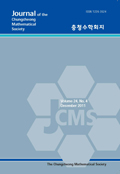상세검색
최근 검색어 전체 삭제
다국어입력
즐겨찾기0KCI등재 학술저널

# SEQUENTIAL PROPERTIES OVER q-COMMUTING ARITHMETIC TABLES

• 등재여부 : KCI등재
• 2019.11
• 475 - 490 (16 pages)
• 4

Let C (q) be the q-commuting arithmetic table of (x + y) n with noncommuting variables. We study sequential properties of diagonal sums of C (q) with various q > 0. One of our results shows that each diagonal sum plays like Fibonacci number with some minor conditions.

1. Introduction

2. Sequential properties on C (q) for q > 0

3. Simpli&#64257;ed q-commuting table